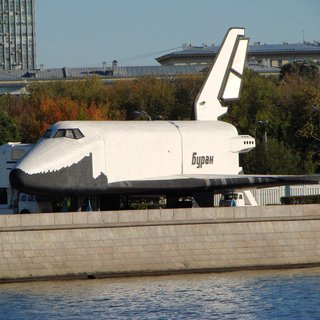OpenCV  3.4.16 Open Source Computer Vision

Next Tutorial: How to enable Halide backend for improve efficiency

## Introduction

In this tutorial you will learn how to use opencv_dnn module for image classification by using GoogLeNet trained network from Caffe model zoo.

We will demonstrate results of this example on the following picture.Buran space shuttle

## Source Code

We will be using snippets from the example application, that can be downloaded here.

#include <fstream>
#include <sstream>
#include <opencv2/dnn.hpp>
#include "common.hpp"
std::string keys =
"{ help h | | Print help message. }"
"{ @alias | | An alias name of model to extract preprocessing parameters from models.yml file. }"
"{ zoo | models.yml | An optional path to file with preprocessing parameters }"
"{ input i | | Path to input image or video file. Skip this argument to capture frames from a camera.}"
"{ framework f | | Optional name of an origin framework of the model. Detect it automatically if it does not set. }"
"{ classes | | Optional path to a text file with names of classes. }"
"{ backend | 0 | Choose one of computation backends: "
"0: automatically (by default), "
"1: Halide language (http://halide-lang.org/), "
"2: Intel's Deep Learning Inference Engine (https://software.intel.com/openvino-toolkit), "
"3: OpenCV implementation }"
"{ target | 0 | Choose one of target computation devices: "
"0: CPU target (by default), "
"1: OpenCL, "
"2: OpenCL fp16 (half-float precision), "
"3: VPU }";
using namespace cv;
using namespace dnn;
std::vector<std::string> classes;
int main(int argc, char** argv)
{
CommandLineParser parser(argc, argv, keys);
const std::string modelName = parser.get<String>("@alias");
const std::string zooFile = parser.get<String>("zoo");
keys += genPreprocArguments(modelName, zooFile);
parser = CommandLineParser(argc, argv, keys);
parser.about("Use this script to run classification deep learning networks using OpenCV.");
if (argc == 1 || parser.has("help"))
{
parser.printMessage();
return 0;
}
float scale = parser.get<float>("scale");
Scalar mean = parser.get<Scalar>("mean");
bool swapRB = parser.get<bool>("rgb");
int inpWidth = parser.get<int>("width");
int inpHeight = parser.get<int>("height");
String model = findFile(parser.get<String>("model"));
String config = findFile(parser.get<String>("config"));
String framework = parser.get<String>("framework");
int backendId = parser.get<int>("backend");
int targetId = parser.get<int>("target");
// Open file with classes names.
if (parser.has("classes"))
{
std::string file = parser.get<String>("classes");
std::ifstream ifs(file.c_str());
if (!ifs.is_open())
std::string line;
while (std::getline(ifs, line))
{
classes.push_back(line);
}
}
if (!parser.check())
{
parser.printErrors();
return 1;
}
CV_Assert(!model.empty());
Net net = readNet(model, config, framework);
net.setPreferableBackend(backendId);
net.setPreferableTarget(targetId);
// Create a window
static const std::string kWinName = "Deep learning image classification in OpenCV";
if (parser.has("input"))
cap.open(parser.get<String>("input"));
else
cap.open(0);
// Process frames.
Mat frame, blob;
while (waitKey(1) < 0)
{
cap >> frame;
if (frame.empty())
{
break;
}
blobFromImage(frame, blob, scale, Size(inpWidth, inpHeight), mean, swapRB, false);
net.setInput(blob);
Mat prob = net.forward();
Point classIdPoint;
double confidence;
minMaxLoc(prob.reshape(1, 1), 0, &confidence, 0, &classIdPoint);
int classId = classIdPoint.x;
// Put efficiency information.
std::vector<double> layersTimes;
double freq = getTickFrequency() / 1000;
double t = net.getPerfProfile(layersTimes) / freq;
std::string label = format("Inference time: %.2f ms", t);
putText(frame, label, Point(0, 15), FONT_HERSHEY_SIMPLEX, 0.5, Scalar(0, 255, 0));
// Print predicted class.
label = format("%s: %.4f", (classes.empty() ? format("Class #%d", classId).c_str() :
classes[classId].c_str()),
confidence);
putText(frame, label, Point(0, 40), FONT_HERSHEY_SIMPLEX, 0.5, Scalar(0, 255, 0));
imshow(kWinName, frame);
}
return 0;
}

## Explanation

Also you need file with names of ILSVRC2012 classes: classification_classes_ILSVRC2012.txt.

Put these files into working dir of this program example.

2. Read and initialize network using path to .prototxt and .caffemodel files
Net net = readNet(model, config, framework);
net.setPreferableBackend(backendId);
net.setPreferableTarget(targetId);
You can skip an argument framework if one of the files model or config has an extension .caffemodel or .prototxt. This way function cv::dnn::readNet can automatically detects a model's format.
3. Read input image and convert to the blob, acceptable by GoogleNet

VideoCapture cap;
if (parser.has("input"))
cap.open(parser.get<String>("input"));
else
cap.open(0);

cv::VideoCapture can load both images and videos.

blobFromImage(frame, blob, scale, Size(inpWidth, inpHeight), mean, swapRB, false);

We convert the image to a 4-dimensional blob (so-called batch) with 1x3x224x224 shape after applying necessary pre-processing like resizing and mean subtraction (-104, -117, -123) for each blue, green and red channels correspondingly using cv::dnn::blobFromImage function.

4. Pass the blob to the network
net.setInput(blob);
5. Make forward pass
Mat prob = net.forward();
During the forward pass output of each network layer is computed, but in this example we need output from the last layer only.
6. Determine the best class
Point classIdPoint;
double confidence;
minMaxLoc(prob.reshape(1, 1), 0, &confidence, 0, &classIdPoint);
int classId = classIdPoint.x;
We put the output of network, which contain probabilities for each of 1000 ILSVRC2012 image classes, to the prob blob. And find the index of element with maximal value in this one. This index corresponds to the class of the image.
7. Run an example from command line
For our image we get prediction of class space shuttle with more than 99% sureness.